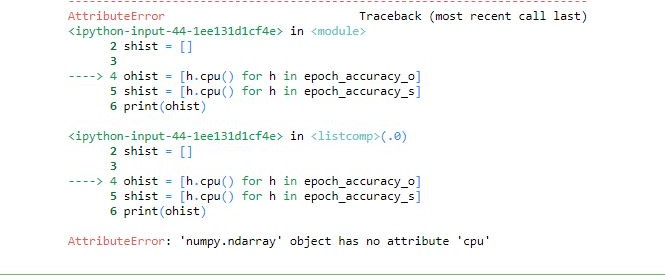# AttributeError: 'numpy.ndarray' object has no attribute 'cpu'

Hi,
I am having a problem in plotting the accuracy of trained model, I adopted the following code for plotting the accuracy from tutorial https://pytorch.org/tutorials/beginner/finetuning_torchvision_models_tutorial.html#model-training-and-validation-code.
Here is the code,

``````ohist = []
shist = []

ohist = [h.cpu().numpy() for h in epoch_accuracy_o]
shist = [h.cpu().numpy() for h in epoch_accuracy_s]
print(ohist)
print(shist)
plt.title("Validation Accuracy vs. Number of Training Epochs")
plt.xlim([1, 20])
plt.ylim([0, 1])
plt.yticks(np.linspace(0, 1, 11))
plt.xticks(np.linspace(0, 20, 21))
plt.xlabel('epoch')
plt.ylabel('accuracy')
plt.grid(linestyle='--')

plt.plot(ohist,label="Pretrained")
plt.plot(shist,label="Scratch")
plt.legend()
plt.show()```
``````

and here is the error I got,After I printed what’s included in h, this is how it looks,

Is there any way I can solve it please?

I’m not sure, but it seems epoch_accuracy_o is a 2d NumPy array. Try this code:
`ohist = [h.cpu()numpy() for h in epoch_accuracy_o]`

Thank you for the quick reply sir.
I tried and it says like

Somehow I changed the `[h.cpu().numpy() for h in epoch_accuracy_o]` into `ohist = [h for h in epoch_accuracy_o]` then it works.

Try this and show here the results:

``````print(epoch_accuracy_o)
print(epoch_accuracy_o.shape)
``````

here h is a numpy array, but it must be pytorch tensor…